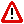To Return to the Prior Page "Click" the Browser Back Button Until the Desired Page Appears

 Prime numbers

Prime numbers are often defined as any integer that has only two factors, which are one, "1," and itself.

The set of prime numbers are the result of the combination of three different "sets" of numbers.  In Unimetry these numbers are often referred to as conventional prime numbers, as opposed to Unimetry's Natural prime numbers,

The sets are:
1.) Par prime numbers;
2.) Par3 prime numbers; and,
3.) The third set is a selected set that is subtracted from the first two sets and is anthropomorphically defined; and, which obfuscates the uniformity of the other two sets, which are determined by the diagonal, "d," of the Pulsoids that underlies seminal motion.

Thank you for your interest.  Prime numbers can be a difficult concept to grasp; should you have any further questions regarding the concept of Prime numbers, please E-mail for assistance.

 E-mail :  Brunardot@Brunardot.comTerms of:  ©Copyright 1999-2015 by Brunardot

000101 0:01a